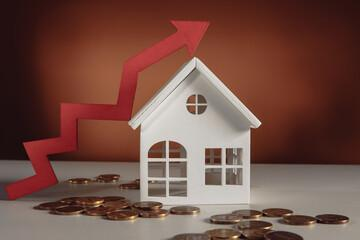Back to Resource Center

# What is Appreciation?What Is Appreciation?

In general, appreciation is the increase in the value of an asset through time. Increased demand or weakening supply, as well as changes in inflation or interest rates, can all contribute to an increase in price. This is the polar opposite of depreciation, which is the gradual loss of value.

How Appreciation Works

An increase in any sort of asset, such as a stock, bond, currency, or real estate, is referred to as appreciation. The phrase capital appreciation, for example, refers to an increase in the value of financial assets such as stocks, which can occur for a variety of reasons, including a company’s improved financial performance. Just because an asset’s worth rises does not indicate its owner is aware of it.

A realisation of the rise occurs when the owner revalues the asset at its higher price on their financial statements. Currency appreciation is another sort of appreciation. In respect to other currencies, the value of a country’s currency can increase or depreciate over time.

How to Calculate the Appreciation Rate

The rate of appreciation is nearly identical to the compound annual growth rate (CAGR). As a result, you divide the ending value by the beginning value, then multiply the result by the number of holding periods to get 1 dividend (e.g. years). Finally, you deduct one from the result. However, in order to determine the appreciation rate, you must first know the investment’s starting worth and its future value. You must also know how long the asset will increase in value.

Sam, for example, purchased a property in 2016 for \$100,000. The value has risen to \$125,000 in 2021. During these five years, the house has appreciated by 25% [(\$125,000 – \$100,000) / \$100,000]. [(\$125,000 / \$100,000)^(1/5) – 1] The compound annual growth rate (or CAGR) is 4.6 percent.

Appreciation vs. Depreciation

Appreciation is also a term used in accounting to describe an increase in the value of an asset recorded on a company’s books. The most typical accounting adjustment to an asset’s value is depreciation, which is a negative adjustment. Certain assets have a proclivity for gain, whereas others deteriorate with time. Assets with a finite useful life, on the whole, depreciate rather than appreciate.

Depreciation is often used as an asset’s economic value depreciates over time, such as when a piece of machinery is used beyond its useful life. While asset appreciation is rare in accounting, assets like trademarks may receive an upward value revision as a result of improved brand recognition. Real estate, stocks, and precious metals are examples of assets purchased with the hope that their value will increase in the future. Automobiles, computers, and physical equipment, on the other hand, gradually lose value as their useful lives progress.

Example of Capital Appreciation

When an investor buys a stock for \$10, the stock pays a \$1 annual dividend, resulting in a 10% dividend yield. The stock is now trading at \$15 per share a year later, and the investor has received a \$1 dividend.

As the stock price rose from its purchase price or cost basis of \$10 to its current market value of \$15, the investor received a \$5 return through capital appreciation. In percentage terms, the increase in stock price resulted in a 50 percent return on capital. In keeping with the original dividend yield, the dividend income return is \$1, corresponding to a 10% return. The total return on the stock is \$6, or 60 percent, when capital appreciation and dividend returns are added together.

Are you interested in learning how to get started investing in real estate with as little as \$2500? Then, you must contact us as soon as possible.

Related Topics to Appreciation: